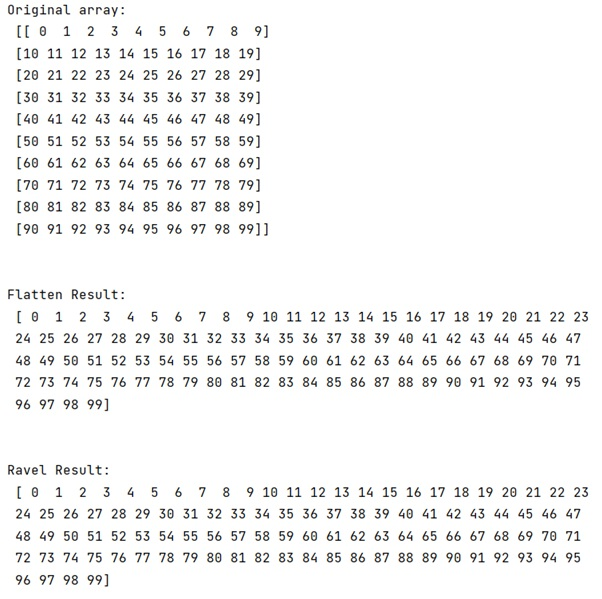# What's the correct and efficient way to flatten NumPy array?

Learn about the correct and efficient way to flatten NumPy array.
Submitted by Pranit Sharma, on March 09, 2023

## Flattening a NumPy Array

Suppose that we are given a 2D NumPy array and we need to make this array flat. We can use different approaches to flatten an array like using flatten() function on a casted list but it is inefficient because we need to cast the array into a list first.

But in NumPy, we have two methods i.e., numpy.flatten() and numpy.ravel(). Both return a 1-d array from an n-d array.

Also, if we do not want to modify the returned 1-d array, it is recommended to use numpy.ravel(), since it does not make a copy of the array, but just returns a view of the array, which is much faster than numpy.flatten.

Let us understand with the help of an example,

## Python code to flatten NumPy array

```# Import numpy
import numpy as np

# Creating a numpy array
arr = np.arange(100).reshape((10,10))

# Display Original array
print("Original array:\n",arr,"\n\n")

# Using arr.flatten
res = arr.flatten()

# Display result
print("Flatten Result:\n",res,"\n\n")

# Using arr.ravel
res = arr.ravel()

# Display result
print("Ravel Result:\n",res)
```

### OutputLanguages: » C » C++ » C++ STL » Java » Data Structure » C#.Net » Android » Kotlin » SQL
Web Technologies: » PHP » Python » JavaScript » CSS » Ajax » Node.js » Web programming/HTML
Solved programs: » C » C++ » DS » Java » C#
Aptitude que. & ans.: » C » C++ » Java » DBMS
Interview que. & ans.: » C » Embedded C » Java » SEO » HR
CS Subjects: » CS Basics » O.S. » Networks » DBMS » Embedded Systems » Cloud Computing
» Machine learning » CS Organizations » Linux » DOS
More: » Articles » Puzzles » News/Updates